1637841600

# How To Implement Navie Bayes From Scratch Algorithm Easily

👉In machine learning, there are primarily four types of classification algorithms. In this essay, I will attempt to describe the Navie Bayes method in depth from the ground up.

⭐️You can see more at the link at the end of the article. Thank you for your interest in the blog, if you find it interesting, please give me a like, comment and share with everyone. Thanks! ❤️

#algorithm

## Buddha Community1637841600

## How To Implement Navie Bayes From Scratch Algorithm Easily

👉In machine learning, there are primarily four types of classification algorithms. In this essay, I will attempt to describe the Navie Bayes method in depth from the ground up.

⭐️You can see more at the link at the end of the article. Thank you for your interest in the blog, if you find it interesting, please give me a like, comment and share with everyone. Thanks! ❤️

#algorithm1596465840

## The Ironic Sophistication of Naive Bayes Classifiers

#### Filtering spam with Multinomial Naive Bayes (From Scratch)

In the first half of 2020 more than 50% of all email traffic on the planet was spam. Spammers typically receive 1 reply for every 12,500,000 emails sent which doesn’t sound like much until you realize more than 15 billion spam emails are being sent each and every day. Spam is costing businesses 20–200 billion dollars per year and that number is only expected to grow.What can we do to save ourselves from spam???

#### Naive Bayes Classifiers

In probability theory and statistics, Bayes’ theorem (alternatively Bayes’s theoremBayes’s law or Bayes’s rule) describes the probability of an event, based on prior knowledge of conditions that might be related to the event.

For example, if the risk of developing health problems is known to increase with age, Bayes’s theorem allows the risk to an individual of a known age to be assessed more accurately than simply assuming that the individual is typical of the population as a whole.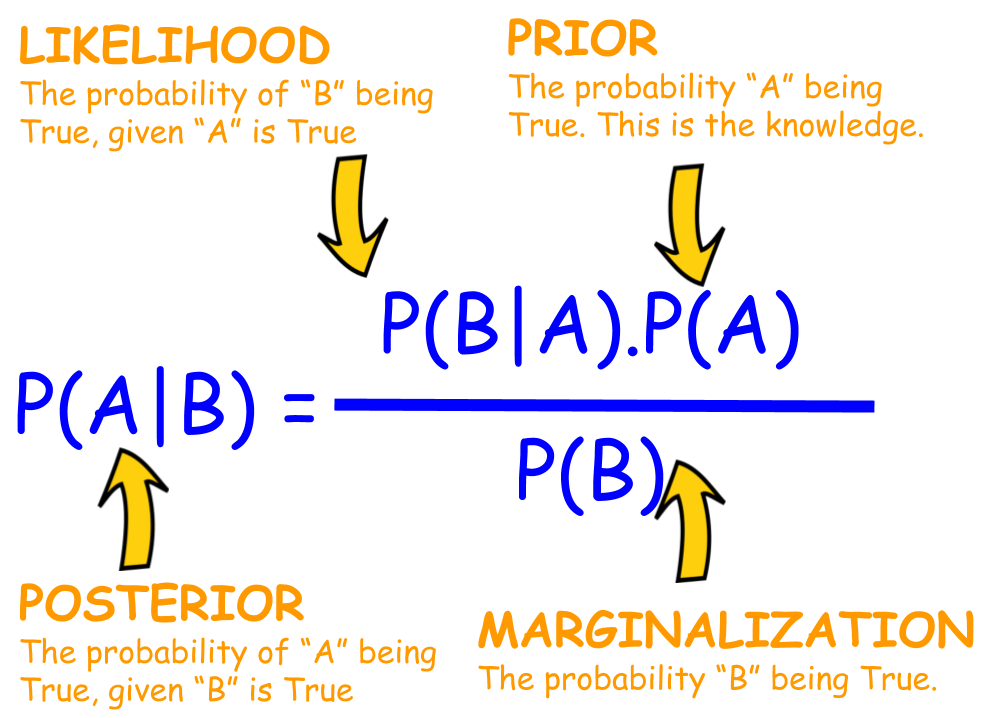Bayes Theorem Explained

#### A Naive Bayes Classifier is a probabilistic classifier that uses Bayes theorem with strong independence (naive) assumptions between features.

• Probabilistic classifier: a classifier that is able to predict, given an observation of an input, a probability distribution over a set of classes, rather than only outputting the most likely class that the observation should belong to.
• Independence: Two events are **independent **if the occurrence of one does not affect the probability of occurrence of the other (equivalently, does not affect the odds). That assumption of independence of features is what makes Naive Bayes naive! In real world, the independence assumption is often violated, but naive Bayes classiﬁers still tend to perform very well.

#naive-bayes-classifier #python #naive-bayes #naive-bayes-from-scratch #naive-bayes-in-python1594944480

## Algorithms from Scratch: Logistic Regression

Contrary to popular belief, I hereby state that Logistic Regression is **NOT **a classification algorithm (on its own) — In fact, Logistic Regression is actually a regression model so don’t be surprised “regression” is present in its naming. Regression analysis is a set of statistical process for estimating the relationships between a dependent variable and one or more independent variables (Source: Wikipedia). With that being stated, Logistic regression is empathetically not a classification algorithm — it does not perform statistical classification — since it is simply estimating the parameters of a logistic model.

Logistic Regression is a statistical model that in its most basic form uses a logistic function to model a binary dependent variable, although many more complex extensions exist. (Source: Wikipedia)

What allows Logistic Regression to be used a classification algorithm, as we so commonly do in Machine Learning, is the use of a threshold (may also be referred to as a cut off or decision boundary), which in-turn will classify the inputs with a probability greater than the threshold as one class and probabilities below the threshold as another class._See _this link** to see how we may approach multiclass classification problem.**Now that’s out of the way, let’s revert our attention back to the purpose of the Algorithms from Scratch series.Link to Github Repository…

## kurtispykes/ml-from-scratch

Permalink Dismiss GitHub is home to over 50 million developers working together to host and review code, manage…

github.com

Note: There are many Machine Learning frameworks with highly optimized code which makes coding Machine learning algorithms from scratch a redundant task in practical settings. However, when we build algorithms from scratch it helps us to gain a deeper intuition of what is happening in the models which may pay high returns when trying to improve our model.

## Linear Regression to Binary Classification

In the last episode of _Algorithms from Scratch: Linear Regression, _I stated “It is usually one of the first algorithms that is learnt when first learning Machine Learning, due to its simplicity and how it builds into other algorithms like Logistic Regression and Neural Networks” — You’ll now see what I meant.How do we go from predicting a continuous variable to Bernoulli variables (i.e. “success” or “failure”)? Well, since the response data (what we are trying to predict) is binary (taking on values 0 and 1), ergo made of only 2 values, we can assume the distribution of our response variable is now from the Binomial distribution — This calls for a perfect time to introduce the Generalized Linear Model (GLM) which was formulated John Nelder and Robert Wedderburn.The GLM model allows for the response variable to have an error distribution other than the normal distribution. In our situation, where we now have a Binomial distribution, by using a GLM model we can generalize linear regression by allowing the linear model to be related to the response variable via a link function and allowing the magnitude of the variance of each measurement to be a function of its predicted value (Source: Wikipedia).

Long story short, Logistic Regression is a special case of the GLM with a binomial conditional response and logit link.

**Halt…**Before going any further we should clear up some statistical terms (In layman’s terms that is):

• **Odds **— The ratio of something happening to something not happening. For instance, the odds of Chelsea FC winning the next 4 games are 1 to 3. The ratio of something happening (Chelsea winning the game) 1 to something not happening (Chelsea not winning the game) 3 can be written as a fraction, 1/3.**Probability **— The ratio of something happening to everything that can happen. Using the example above, the ratio of something happening (Chelsea winning) 1 to everything that can happen (Chelsea winning and losing) 4 can also be written as a fraction 1/4, which is the probability of winning — The probability of losing is therefore 1–1/4 = 3/4.

Probabilities range between 0 and 1, whereas odds are not constrained to be between 0 and 1, but can take any value from 0 to infinity.We can derive the odds from the probability by dividing the ratio of the probability of winning (using our example that would be 1/4) by the ratio of the probability of losing (3/4) which gives us 1/3 — the odds. See Figure 1 for how we can express that mathematically.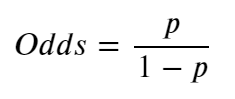Figure 1: Odds derived from the probability.

If Chelsea were a bad Football Team (Unimaginable, I know) the odds would be against them winning therefore ranging between 0 and 1. However, since we all know that Chelsea is one of the greatest teams in the world (definitely the best in London without a doubt), as a consequence, the odds in favour of Chelsea winning will be between 1 and infinity. The asymmetry makes it difficult to compare the odds for or against Chelsea winning so we take the log of the odds to make everything symmetrical._Figure 1 _shows us that we can calculate the odds with probabilities, that being the case, we can also calculate the log of the odds using the formula presented in Figure 1. The log of the ratio of the probabilities is called the logit function and forms the basis of Logistic Regression. Let’s understand this better by considering a logistic model with given parameters and see how the coefficients can be estimated from the data.

#algorithms-from-scratch #algorithms1594944360

## Algorithms From Scratch: Linear Regression

Linear Regression is a popular linear Machine Learning algorithm for regression-based problems. It is usually one of the first algorithms that is learnt when first learning Machine Learning, due to its simplicity and how it builds into other algorithms like Logistic Regression and Neural Networks. In this story we are going to implement it from scratch so that we can build our intuition about what is going on within a Linear Regression model.Link to Github Repo…

## kurtispykes/ml-from-scratch

Permalink Dismiss GitHub is home to over 50 million developers working together to host and review code, manage…

github.com

Note: There are many frameworks with highly optimized code such as Scikit-Learn, Tensorflow and PyTorch, therefore it is usually unnecessary to build your own algorithm from scratch. However, it serves a good purpose to our intuition of what is happening within the model when we build it from scratch and this helps when we attempt to improve our models performance.

A linear model is an algorithm that makes a prediction by simply computing a weighted sum of the input features plus a bias term (also referred to as the intercept term). Taking that into perspective, what we are doing when we use a linear regression model is we hope to explain the relationship between a dependent variable (i.e house price) and one or more independent variables (i.e. location, bedrooms, area, etc).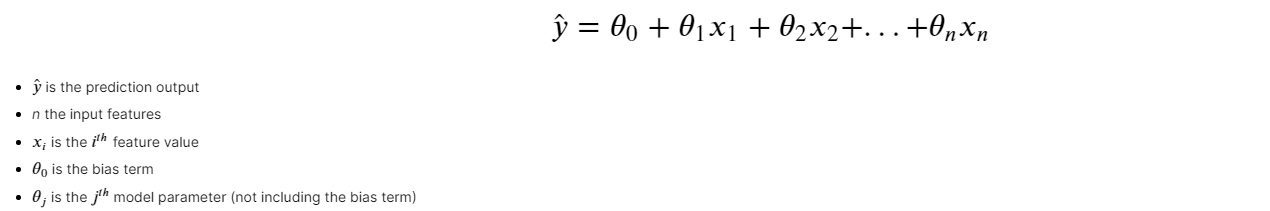Figure 1: Multiple Linear Regression

When we train a model we are attempting to set the parameters to get a line that best fits the training data. Therefore, when we train a Linear Regression model we are trying to find the value of theta that best minimizes the cost function. The most common cost function of a regression models is the RMSE, however, it is much easier to minimize the MSE as it leads to the same result.

## Creating the Model

If you have never written a Machine Learning algorithm from scratch, I greatly encourage you to do so. John Sullivan wrote a very useful story titled 6 Steps To Write Any Machine Learning Algorithm From Scratch: Perceptron Case Study_ w_hich is the best advice I have managed to find on the internet about writing algorithms from scratch.Chunking the Algorithm

1. Randomly initialize parameters for the hypothesis functionCalculate the Partial Derivative (Read more about this here)Update parametersRepeat 2–3 for n number of iterations (Until cost function is minimized otherwise)Inference

ImplementationFor this section, I will be leveraging 3 Python packages. NumPy for Linear algebra, Scikit-Learn which is a popular Machine Learning framework and Matplotlib to visualize our data.

``````import numpy as np
import matplotlib.pyplot as plt
from sklearn.datasets import make_regression
from sklearn.linear_model import LinearRegression
from sklearn.model_selection import train_test_split
from sklearn.metrics import mean_squared_error
``````

First, we need a dataset. To do this I will `sklearn.datasets.make_regression` which allows you to generate a random regression problem — see Documentation. Next, I will split my data into training and test sets with `sklearn.model_selection.train_test_split` — Documentation.

``````# creating the data set
X, y = make_regression(n_samples=100, n_features=1, n_targets=1, noise=20, random_state=24)

# splitting training and test
X_train, X_test, y_train, y_test = train_test_split(X, y, train_size=0.75, random_state=24)
``````

Lets use `matplotlib.pyplot` to see how our data looks — Documentation.

``````# visualize
plt.scatter(X, y)
plt.show()
``````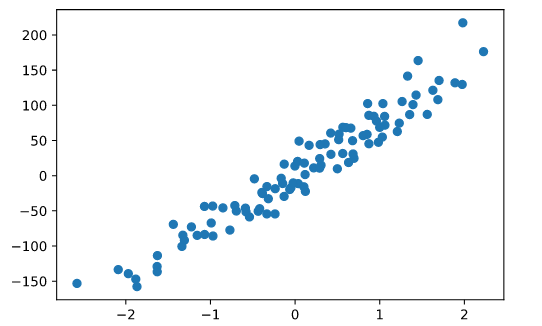Figure 2: Our generated regression problem

Now we can begin our implementation of Linear Regression. The first step of our chunk is to randomly initialize parameters for our hypothesis function.

``````def param_init(X):
"""
Initialize parameters for linear regression model
__________________
Input(s)
X: Training data
__________________
Output(s)
params: Dictionary containing coefficients
"""
params = {} # initialize dictionary
_, n_features = X.shape # shape of training data

# initializing coefficents to 0
params["W"] = np.zeros(n_features)
params["b"] = 0
return params
``````

Excellent. Next we want to calculate the partial derivatives and update our parameters. We do this using a very important Machine Learning algorithm called Gradient Descent. Ergo, we can implement steps 2–4 with Gradient Descent.

#algorithms-from-scratch #machine-learning #data-science #algorithms1596737040

## Algorithms From Scratch: Support Vector Machine

A popular algorithm that is capable of performing linear or non-linear classification and regression, Support Vector Machines were the talk of the town before the rise of deep learning due to the exciting kernel trick — If the terminology makes no sense to you right now don’t worry about it. By the end of this post you’ll have an good understanding about the intuition of SVMs, what is happening under the hood of linear SVMs, and how to implement one in Python.

To see the full Algorithms from Scratch Series click on the link below.

## Algorithms From Scratch - Towards Data Science

Read writing about Algorithms From Scratch in Towards Data Science. A Medium publication sharing concepts, ideas, and…

towardsdatascience.com

## Intuition

In classification problems the objective of the SVM is to fit the largest possible margin between the 2 classes. On the contrary, regression task flips the objective of classification task and attempts to fit as many instances as possible within the margin — We will first focus on classification.

If we focus solely on the extremes of the data (the observations that are on the edges of the cluster) and we define a threshold to be the mid-point between the two extremes, we are left with a margin that we use to sepereate the two classes — this is often referred to as a hyperplane. When we apply a threshold that gives us the largest margin (meaning that we are strict to ensure that no instances land within the margin) to make classifications this is called Hard Margin Classification (some text refer to this as Maximal Margin Classification).

When detailing hard margin classification it always helps to see what is happening visually, hence Figure 2 is an example of a hard margin classification. To do this we will use the iris dataset from scikit-learn and utility function `plot_svm()` which you can find when you access the full code on github — link below.

## kurtispykes/ml-from-scratch

Permalink Dismiss GitHub is home to over 50 million developers working together to host and review code, manage…

github.com

Note: This story was written straight from jupyter notebooks using python package `_jupyter_to_medium_` — for more information on this package click here— and the committed version on github is a first draft hence you may notice some alterations to this post.

``````import pandas as pd
import numpy as np
from sklearn.svm import LinearSVC
from sklearn.preprocessing import StandardScaler
import matplotlib.pyplot as plt
%matplotlib inline
# store the data

# convert to DataFrame
df = pd.DataFrame(data=iris.data,
columns= iris.feature_names)

# store mapping of targets and target names
target_dict = dict(zip(set(iris.target), iris.target_names))

# add the target labels and the feature names
df["target"] = iris.target
df["target_names"] = df.target.map(target_dict)

# view the data
df.tail()
``````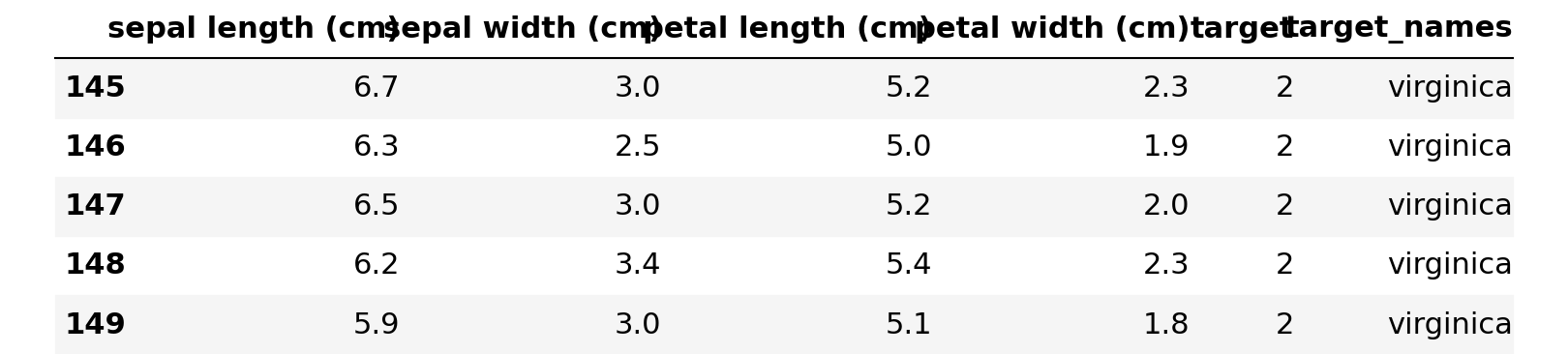Figure 1: Original Dataset

``````# setting X and y
X = df.query("target_names == 'setosa' or target_names == 'versicolor'").loc[:, "petal length (cm)":"petal width (cm)"]
y = df.query("target_names == 'setosa' or target_names == 'versicolor'").loc[:, "target"]

# fit the model with hard margin (Large C parameter)
svc = LinearSVC(loss="hinge", C=1000)
svc.fit(X, y)

plot_svm()
``````

#programming #machine-learning #data-science #artificial-intelligence #algorithms-from-scratch #algorithms